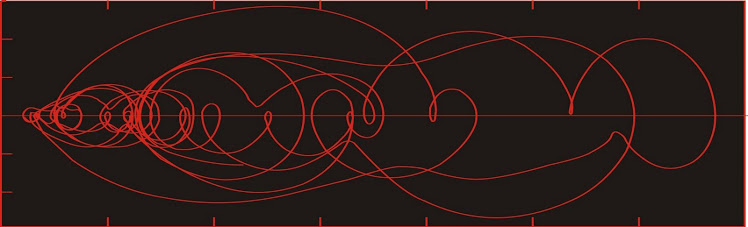## Dust flux, Vostok ice coreTwo dimensional phase space reconstruction of dust flux from the Vostok core over the period 186-4 ka using the time derivative method. Dust flux on the x-axis, rate of change is on the y-axis. From Gipp (2001).

## Thursday, September 22, 2011

### Information theoretic approaches to characterizing complex systems, part 4: Formalizing a method for optimizing window lengths for probability density diagrams from phase space

When the market looks like it does today, it is better to think about other things.

Some time ago we began looking at the problem of determining the optimum window over which the probability density of the phase space should be calculated. The problem is essentially one of optimization, where if the window is too short the probability density plot will not be accurately represented, but if it is too long, then interesting features may be smoothed out.

Once again we look at a > 2 million year proxy for the strength of the Himalayan paleomonsoon (top figure).

Reconstructing a phase space portrait over small (say 30 thousand years) intervals gives us highly variable results because of the variability in the dynamic behaviour of the system over comparatively short timeframes. As we have seen elsewhere, many of these complex climatic systems are characterized by intervals during which the state space is confined within a comparatively small area of Lyapunov stability, interspersed with intervals during which the system evolves rapidly towards another area of stability.

While confined within an LSA, the probability density will consist of a few large values spread over a small area. The entropy (in an information theory sense) will be small.

When the system is evolving towards a new LSA, the probability density will consist of many small values spread over a large area. The entropy will be large.

Successive values of entropy calculated over small time windows will show a lot of variability. Some of that variability will be due to secular changes in the complexity of the system, and some will be due to the granularity of our observations. If we start choosing longer time windows, we get tend to get both episodes of stability and bifurcation within each window, so the effects of granularity ideally vanish and only the secular variations remain.

Variability declines as the window length increases from 30 ky (thousand years) to 150 ky. Now, each of the above graphs consists of a string of data, so we can do better than eyeballing a comparison.

The methodology proposed then is to normalize each of the strings of data above, and then compare the zero-lag cross-correlation of the entropy of two successive window-lengths to the zero-lag autocorrelation of the entropy observed in shorter of the two windows.

For instance, in the figure above, the entropy observed over 30-ky windows varies from about 1.5 to 3.5. We normalize the data by dividing the difference between each observation and the mean of the series by its standard deviation   i.e. x(norm)= (x - mean)/(standard deviation)

We similarly normalize the entropy values for the 60-ky window. The zero-lag cross-correlation will have a lower value than the zero-lag autocorrelation--but how much lower is a measure of how different the two curves are. We find that the ratio of the two values improves as we look at longer windows, as below.

The graph converges in the general direction of the value of 1 as the window gets longer. A value of one would imply perfect correlation between the two entropy functions. So we choose the window length for which the zero-lag cross-correlation is sufficiently high for our purposes. In the example above, I would find that the 150 ky window is sufficient.

For the late Quaternary, a window length of 150 ky also appears suitable.

I'm pretty sure that the different rates at which the cross-correlations approach 1 in the Late and Early Quaternary paleomonsoon proxy are telling us something about the dynamics of the system over these two intervals--but I'm not yet sure what.